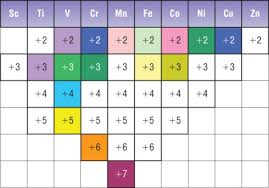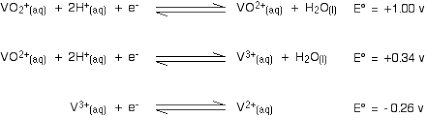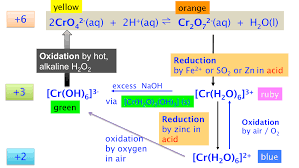# Edexcel Chemistry - Topic 15: Inorganic Chemistry II - Transition Metals

• Created by: Ryan C-S
• Created on: 07-04-18 11:25

## General Properties of Transition Metals

• A transition metal is a metal that forms one or more stable ions which have an incompletely filled d orbital
• When forming ions the 4s subshell loses electrons before the 3d subshell which means that incompletely filled d orbitals can arise
• Successive ionisation energies for the elements Sc to Zn show a gradual increase until all the 4s and 3d electrons have been removed, then there is a big jump as electrons from a new shell are removed
• Chromium and Copper have configurations with 4s1 3d5 or 4s1 3d10 as these arrangements are more stable due to equal charge distribution
• Scandium is not a transition metal as it can only form a +3 ion with an empty d orbital
• Zinc is not a transition metal as it can only form a +2 ion with a complete d orbital
Therefore both Scandium and Zinc don't meet the definition of a transition metal and lack certain properties that are characteristic of transition metals such as:
• Complex Ion formation
• Formation of Coloured Ions and Compounds
• Variable Oxidation States
• Catalytic Activity
1 of 22

## Complex Formation

• A complex is a central metal ion surrounded by ligands
• A ligand is an atom, ion or molecule which can donate a lone pair of electrons
• Dative/Co-ordinate bonding is involved in complex formation as the covalent bond comes from the shared pair of electrons
• Coordination number is the number of co-ordinate bonds formed to a central metal ion
• Ligands can be:
Monodentate (e.g. H2O, NH3) which can form one coordinate bond per ligand
Bidentate (e.g. [C2O4]2-) which have two atoms with lone pairs and can form two coordinate bonds per ligand
Multidentate (e.g. [EDTA]4-) which can form multiple coordinate bonds per ligand2 of 22

## Shapes of Complex Ions

• The shapes of complex ions follow the VSEPR rules
• Transition metals generally form octahedral complexes with small ligands (e.g. H2O or NH3) and tetrahedral complexes with larger ligands (e.g. Cl-)
• Silver ions generally form linear complexes e.g. [Ag(NH3)2]+
• Cisplatin is a square planar platinum complex that is used as an anticancer drug. The complex consists of two chloride ions and two ammonia ligands that are on the same (cis) side of the molecule to enable the drug to bind to DNA and stop the replication of cancerous cells
• Iron(II) in haemoglobin enables oxygen to be transported in the blood. Haem is an Iron(II) complex with a multidentate ligand. Carbon monoxide can form a strong coordinate bond with haemoglobin and replaces oxygen in a ligand exchange reaction hence why Carbon Monoxide is toxic.3 of 22

## Coloured Ions

• Colour arises from electronic transitions from the ground state to excited states between different d orbitals
• A portion of visible light is absorbed to promote d electrons to higher energy levels. The light that is not absorbed is transmitted to give the substance colour (e.g. A solution appears blue if it absorbs orange light)
•4 of 22

## Changing Colour

• Colour Changes arise from changes in:
Oxidation State
Co-ordination Number
Ligand
• Changing a ligand or the coordination number will alter the energy split between the
d orbitals, changing the energy and hence the frequency of light absorbed

[Cu(H2O)6]2+ + 4Cl- --> [CuCl4]2- + 6H2O
*In this equation both ligand and co-ordination number are changing

[Co(H2O)6]2+ + 6NH3 --> [Co(NH3)6]2+ + 6H2O
*In this equation only ligand is changing

• The Scandium ion hasn't got any d electrons left to move around so there is not an energy transfer equal to that of visible light so no colour is displayed
• The Zinc ion has a full d shell so there is no space for electrons to transfer and so there is not an energy transfer avaliable equal to that of visible light so no colour is displayed
5 of 22

## Spectrophotometry

• Spectrophotometry is a method to measure how much a chemical substance absorbs light
• If visible light of increasing freuency is passed through a sample of a coloured complex ion, some of the light is absorbed
• The amount of light absorbed is proportional to the concentration of the absorbing species

Method

• Add an appropriate ligand to intensify colour
• Make up solutions of known concentrations of ligand
• Measure absorption or transmission
• Plot a graph of the results or a calibration curve
• Measure the absorbtion for unknown and compare
6 of 22

## Variable Oxidation States

• Transition elements form ions by losing the 4s electrons before the 3d electrons
• Transition metals form variable oxidation states because they are:
Able to donate and receive electrons
Able to oxidise and reduce
• This is because the ions contain partially filled sub-shells of d electrons that can easily gain or lose electrons. The energy differences between oxidation states are small
• Relative stability of +2 state with respect to +3 state increases across the period
• Compounds with high oxidation states tend to be oxidising agents (e.g. MnO4-)
• Compounds with low oxidation states tend to be reducing agents (e.g. Fe2+)7 of 22

• Vanadium exists at four main oxidation states
[VO2]+ = Oxidation State +5 (yellow solution)
[VO]2+ = Oxidation State +4 (blue solution)
[V]3+ = Oxidation State +3 (green solution)
[V]2+ = Oxidation State +2 (violet solution)
• Addition of zinc to Vanadium in acidic solution will reduce vanadium down through each successive oxidation state, and the colour would change through each of the colours
• As zinc has a more negative electrode potential (E = -0.76V) than all the vanadium half equations, zinc will reduce down to [V]2+
• Tin metal (E = -0.14V) will only reduce the vanadium to [V]3+
Zn2+  + 2e-   --> Zn      E = -0.76V
Sn2+  + 2e-   --> Sn      E = -0.14V8 of 22

## Reducing Chromium

• Cr3+ (green) and Cr2+ (blue) are formed by reduction of [Cr2O7]2- (orange) by the strong reducing agent zinc in (HCl) acid solution
• Fe2+ is a less strong reducing agent and will only reduce the dichromate to Cr3+
[Cr2O7]2- + 3Zn + 14H+ --> 2Cr3+ + 7H2O + 3Zn2+
[Cr2O7]2- + 4Zn + 14H+ --> 2Cr2+ + 7H2O + 4Zn2+
• The Zinc and dichromate are kept under a hydrogen atmosphere to reduce it to Cr2+ because the O2 in air will oxidise Cr2+ up to Cr3+
• The Cr2+ state can be stabilised by forming a stable complex ion with a ligand such as the ethanoate ion. If the blue Cr2+ ions are bubbled through sodium ethanoate, a stable red precipitate of chromium(II) ethanoate is formed
• The electrode potential of Iron(II) (E = +0.75V) is between the electrode potential of Cr3+
(E = +1.33V) and Cr2+ (E = -0.41V) so will only reduce chromium down to Cr3+
• Zinc has a more negative electrode potential than all chromium half equations so Zinc will reduce chromium down to Cr2+
[Cr2O7]2- + 6e- + 14H+ --> 2Cr3+ + 7H2O  E = +1.33V
Fe3+  + e- --> Fe2+  E = +0.75V
Cr3+  + e- --> Cr2+   E = -0.41V
Zn2+ + 2e- --> Zn     E = -0.76V
9 of 22

## Oxidising Chromium

• Transition metals in low oxidation states are more easily oxidised in alkaline solutions than in acidic solutions
• It is easier to remove an electron from a negatively charged ion
• The chromium ions can be oxidised by using an oxidising agent such as hydrogen peroxide

[Cr(H2O)6]3+(aq) --> [Cr(OH)6]3-(aq)  conditions: excess Sodium Hydroxide
[Cr(OH)6]3-(aq) --> [CrO4]2-(aq)   conditions: Hydrogen Peroxide

Red: H2O2 + 2e- --> 2OH-
Ox: [Cr(OH)6]3- + 2OH- --> [CrO4]2- + 3e- + 4H2O
2[Cr(OH)6]3- + 3H2O2 --> 2[CrO4]2- + 2OH- + 8H2O

• To balance half equations in aqueous solutions the following steps are followed:
Add OH- to both sides to cancel out H+
10 of 22

## Chromate/Dichromate Equilibrium

• The Chromate ([CrO4]2-) and Dichromate ([Cr2O7]2-) ions can be converted from one to the other by the following equilibrium reaction:

2[CrO4]2- + 2H+  [Cr2O7]2- + H2O
(yellow solution)      (orange solution)

• Addition of acid will, by application of Le Chatelier's Principle, push the equilibrium to the dichromate
• Addition of alkali will remove the H+ ions and, by application of Le Chatelier's Principle, push the equilibrium to the chromate
• This is not a redox reaction as both the chromate and dichromate ions have an oxidation number of +6. This is an acid-base reaction
11 of 22

## Chromium Reactions Summary12 of 22

## Reactions of Aqueous Inorganic Compounds

• Lewis acid: electron pair acceptor
• Lewis base: electron pair donator
• In the formation of complex ions, the ligand is the Lewis base because it is donating a pair of electrons in the dative covalent bond and the metal ion is the Lewis acid
• Metal aqua ions are formed in aqueous solution
[M(H2O)6]2+ ions limited to M = Fe (green), Co (pink) and Cu (blue)
[M(H2O)6]3+ ions limited to M = Cr (ruby) and Fe (violet)
• In solutions Cr(III) appears green and Fe(III) appears yellow/brown due to hydrolysis reactions. The ruby and violet colours are only seen in solid hydrated salts of these complexes
• The following equilibria happen in aqueous solutions of metal ions:
[M(H2O)6]2+ + H2O  [M(H2O)5(OH)]+ + H3O+
[M(H2O)6]3+ + H2O  [M(H2O)5(OH)]2+ + H3O+
• The equilibria lead to generation of acidic solutions with M3+ ions and very weakly acidic solutions with M2+ ions.
• The acidity of [M(H2O)6]3+ is greater than that of [M(H2O)6]2+ in terms of the greater polarising power of the 3+ metal ion. The greater the polarising power, the more strongly it attracts the water molecule. This weakens the O-H bond so it breaks more easily.
13 of 22

## Reactions with Limited Alkalis

• The bases OH- and ammonia when in limited amounts form the same hydroxide precipitates
• They form in deprotonation acid-base reactions
M(OH)2(H2O)4(s): Cu (blue ppt), Co (blue ppt), Fe(II) (green ppt)
M(OH)3(H2O)3(s): Cr(III) (green ppt), Fe(III) (brown ppt)

[Cu(H2O)6]2+(aq) + 2OH-(aq) --> Cu(H2O)4(OH)2(s) + 2H2O(l)
[Co(H2O)6]2+(aq) + 2NH3(aq) --> Co(H2O)4(OH)2(s) + 2NH4+(aq)
[Cr(H2O)6]3+(aq) + 3OH-(aq) --> Cr(H2O)3(OH)3(s) + 3H2O(l)
[Fe(H2O)6]3+(aq) + 3NH3(aq) --> Fe(H2O)3(OH)3(s) + 3NH4+(aq)

• The reaction can happen step wise removing one proton at a time:

[Cr(H2O)6]3+(aq) + OH-(aq) --> [Cr(H2O)5(OH)]2+(aq) + H2O(l)
[Cr(H2O)5(OH)]2+(aq) + OH-(aq) --> [Cr(H2O)4(OH)2]+(aq) + H2O(l)

• In these reactions the NH3 and OH- ions act as Bronsted-Lowry bases, accepting a proton
14 of 22

## Reactions with Excess Alkalis

• With excess NaOH, the Cr hydroxide precipitate dissolves. Cr becomes [Cr(OH)6]3-(aq) (green solution)
• The hydroxide prexipitates are amphoteric as they react and dissolve in both acids and bases

Cr(H2O)3(OH)3(s) + 3OH-(aq) --> [Cr(OH)6]3-(aq) + 3H2O(l)
Cr(H2O)3(OH)3(s) + 3H+(aq) --> [Cr(H2O)6]3+(aq)

• With excess NH3, ligand substitution reactions occur with Cu, Co and Cr and their precipitates dissolves. NH3 acts as a Lewis base, donating an electron pair
• The ligands NH3 and H2O are similar in size and are uncharge. Ligand exchange occurs without a change of coordination number for Co and Cr. The substituion however may be incomplete as in the case with Cu.
• Cr becomes [Cr(NH3)6]3+ (purple solution)
• Co becomes [Co(NH)6]3+ (pale yellow solution) - Co complex is oxidised to +3 in air
• Cu becomes [Cu(NH3)4(H2O)2]2+ (deep blue solution)

Cr(OH)3(H2O)3(s) + 6NH3(aq) --> [Cr(NH3)6]3+(aq) + 3H2O(l) + 3OH-(aq)
Cu(OH)2(H2O)4(s)) + 4NH3(aq) --> [Cu(NH3)4(H2O)2]2+(aq) + 2H2O(l) + 2OH-(aq)

15 of 22

## Ligand Exchange Reactions with Chloride Ions

• Addition of a high concentration of chloride ions (from conc HCl or saturated NaCl) to an aqueous ion leads to a ligand substitution reaction.
• The Cl- ligand is larger than the uncharged H2O and NH3 ligands so therefore ligand exchange can involve a change of coordination number
• Addition of conc HCl to aqueous ions of Cu lead to change in coordination number from 6 to 4.
• [CuCl4]2- forms a yellow/green solution and is tetrahedral in shape

[Cu(H2O)6]2+ + 4Cl- --> [CuCl4]2- + 6H2O

*If solid copper chloride (or any other metal) is dissolved in water it forms the aqueous
[Cu(H2O)6]2+ complex and not the chloride [CuCl4]2- complex

16 of 22

## Stability of Complexes

• The Chelate Effect occurs when the substitution of monodentate ligands with a bidentate or multidentate ligand leads to a more stable complex
• This effect can be explained in terms of positive entropy change in these reactions as more molecules of products than reactants are produced

[Cu(H2O)6]2+(aq) + EDTA4-(aq) --> [Cu(EDTA)]2-(aq) + 6H2O(l)

• The copper complex ion has changed from having monodentate ligands to a multidentate ligand
• There is an increase in entropy of the system as there are more moles of products than reactants (from 2 to 7) creating more disorder
• The stability of the EDTA complexes has many applications:
It can be added to rivers to remove poisonous heavy metal ions
It can be added to shampoos to remove calcium ions present in water, helping with lathering
17 of 22

## Ethane-1-2-diamine

• Ethane-1-2-diamine is a common bidentate ligand

[Co(NH3)6]2+ + 3NH2CH2CH2NH2 --> [Co(NH2CH2CH2NH2)3]2+ + 6NH3

• This reaction has an increase in entropy because of the increase in moles from 4 to 7 in the reaction. ΔSsystem is positive.
• Its enthalpy change ΔH (and ΔSsystem) is close to zero as the number of dative covalent and type (Nitrogen to metal coordinate bonds) are the same so the energy required to break and make bonds will be the same
• Therefore ΔStotal will be positive and the complex formed is stable
• Ethane-1-2-diamine, as a base, can also carry out the deprotonation reaction (similar to those of ammonia) forming hydroxide precipitates.

2[Al(H2O)6]3+(aq) + 3NH2CH2CH2NH2(aq) --> 2Al(H2O)3(OH)3(s) + 3 [NH3CH2CH2NH3]2+(aq)

18 of 22

## Catalysts

• Catalysts increase reaction rates without getting used up by providing an alternative route with a lower activation energy
• Transition metals and their compounds can act as heterogenous and homogenous catalysts
• A heterogenous catalyst is in a different phase from the reactants
• A homogenous catalyst is in the same phase as the reactants
• Catalysts provide economic benefits for a reaction as they require less energy to cause a reaction to occur and so lower energy costs for Industries
19 of 22

## Heterogenous Catalysts

• Heterogenous catalysts are usually solids whereas the reactants are gaseous or in solution e.g. V2O5 in the Contact Process
• The reaction occurs at the surface of the catalyst on the active sites
• Adsorbtion of reactants at active sites on the surface may lead to catalytic action. By adsorbing onto the surface of a metal, the bonds within the reactant become weaker and the molecules are held in a more reactive configuration.
There will therefore be a higher concentration of reactants at the solid surface leading to a higher collision frequency
• Transition metals can use the 3d and 4s electrons of atoms on the metal surface to form weak bonds to the reactants
• The strength of adsorbtion helps to determine the effectiveness of the catalytic activity
Some metals (e.g. W) have too strong adsorbtion and so the products cannot be released
Some metals (e.g. Ag) have too weak adsorbtion and so the reactants do not adsorb in high enough concentration
• Nickel and Platinum have about the right strength and are most useful as catalysts
• Increasing the surface area of a solid catalyst improves its effectiveness by increasing the area by which reactions can occur on
• Heterogenous catalysts can be filtered off and are easy to seperate from products
20 of 22

## Homogenous Catalysts

• When a catalyst and reactants are in the same phase, the reaction proceeds through an intermediate species
• The intermediate will have a different oxidation state to the original transition metal. At the end of the reaction, the original oxidation state will reoccur
• Transition metals can act as homogenous catalysts because they can form various oxidation states
• They can donate and receive electrons and are able to oxidise and reduce due to the ions containing a partially filled sub-shell of d electrons that can easily gain/lose electrons
• The reaction between I- and [S2O8]2- is catalysed by Fe2+ or Fe3+.
[S2O8]2- + 2I- --> 2[SO4]2- + I2
• The reaction is slow because the reaction needs collision between two negative ions so repulsion between these ions hinders this - meaning a high activation energy is required
• For a substance to act as a homogenous catalyst, its electrode potential must lie in between the electrode potentials of the two reactants so it can reduce the reactant with the more positive electrode potential and oxidise the reactant with the more negative electrode potential
Stage 1: [S2O8]2- + 2Fe2+ --> 2[SO4]2- + 2Fe3+
Stage 2: 2I- + 2Fe3+ --> 2Fe2+ + I2
21 of 22

## Catalytic Converters

• Catalytic converters remove CO, NOx and unburned hydrocarbons (e.g. octane) from exhaust gases and turn them into 'harmless' CO2, N2 and H2O

2 CO + 2 NO --> 2 CO2 + N2
C8H18 + 25 NO --> 8 CO2 + 12.5 N2 + 9 H2O

• Catalytic converters are heterogenous catalysts consisting of a ceramic honeycomb coated with a thin layer of Platinum, Palladium or Rhodium - to give a larger surface area

Steps in Heterogenous Catalysis in a Catalytic Converter

• CO and NO molecules form bonds with atoms at active sites on the surface of the catalyst - adsorbed onto the surface
• As a result, the bonds in the reactants are weakened and break
• New bonds form between reactants held close together on the catalysts surface
• Desorption of CO2 and N2 product molecules from the catalysts surface
22 of 22